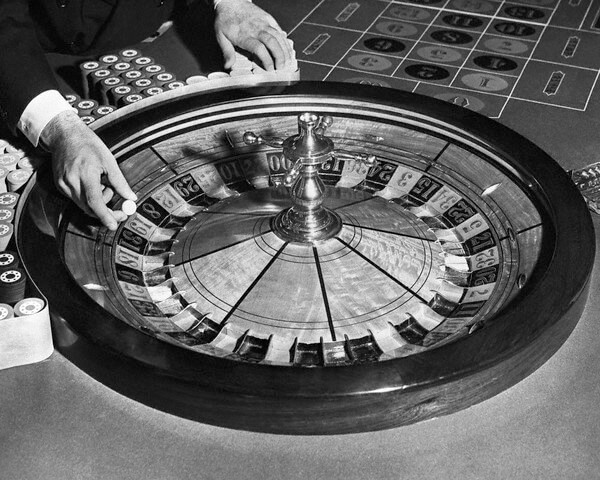The theoretical expectation (frequency) of an event is not the same as the probability of that event.

For example since the are 2 colors at roulette (ignoring the zero), the expectation of the appearance of at least one black in 2 spins is 1/2+1/2=1 or 100% – according to perfect distribution of outcomes.
But the actual probability of having at least one black number in 2 spins is only
1-(1/2*1/2)=0,75 or 75%.

The expectation of an event is usually higher than the probability of the same event.

The expectation is useful in designing our roulette strategies, while the probability is useful in deciding our bankroll. Expectation describes what should happen (in a perfect world or in the long run). Probability describes the certainty of this event happening.

It is very important to understand that the expectation of an event is something different from the probability of that event.